Courses
Courses for Kids
Free study material
Free LIVE classes
More

# NCERT Solutions for Class 10 Maths Chapter 11 - ConstructionsLIVE
Join Vedantu’s FREE Mastercalss

## NCERT Solutions for Class 10 Maths Chapter 11 Constructions - Free PDF

CBSE Class 10 Maths Chapter 11 is based on the construction of different geometric figures. This chapter focuses on teaching how to draw dividing points for line segments and closed figures. This fundamental chapter needs the complete study material for proper preparation. This material should contain the solutions for Class 10 Maths Constructions exercises.

The questions in the exercises have been solved by the subject experts of Vedantu by following the latest CBSE syllabus and guidelines. It will help students to figure out the ideal methods of approaching these fundamental problems and use the geometric concepts explained in this chapter perfectly.

NCERT Solutions for Class 10 Chapter 11, Constructions is well crafted by subject-matter experts in Vedantu. They have developed the NCERT Solutions as per the latest syllabus set by CBSE board. Vedantu also provides relevant notes for the Maths NCERT Solutions Class 10 to give a better understanding of the concept.

You can download the free pdf format of the NCERT Solutions Chapter 11 from Vedantu’s official website. NCERT Solutions for other subjects for other classes are also available on Vedantu. If you have any queries relating to the concepts, you can reach out to our experienced teachers.

## Significance of CBSE Class 10 Maths Constructions Exercise Solutions

This chapter holds immense importance in the Class 10 Maths syllabus as it teaches how to draw different points and figures. The stepwise presentation of the process of drawing such geometric figures and points in the book will become easier to follow when students have the right study material.

This material must contain the NCERT solutions for all the exercises included in this chapter. The exercise problems have been compiled by the CBSE board to check the preparation and comprehension levels of the students. This is where the solutions framed by the experts for all the questions in this chapter will be very useful.

Once a section is done, students will proceed to solve the exercise questions. The solutions to these questions can be referred to find accurate answers. They can check their answers and find out where they need to focus more. Hence, the NCERT solutions for Class 10 Maths Construction can be used as an assessment tool for the preparation of this subject.

## How to use Class 10 Maths Construction Solutions?

Focus on the theory part of the chapter. Use the hands-on method to copy what the textbook is instructing you to do. Follow the steps and achieve what this chapter explains in a section. Once done, practice the process repeatedly.

When you are done practising, proceed to the respective exercise to solve the given problems. Refer to the solutions here and resolve your doubts. Focus on how the experts have skilfully used the chapter theorems and fundamental geometric concepts to draw such figures and answered the exercise questions.

Now, practice following the methods and steps mentioned in the solutions to make the exercise problems easily comprehensible. This is how you can brilliantly use the solutions for all the exercises in this chapter.

In this chapter, you will learn the concept of determining a point dividing a line segment internally given a ratio, construction of similar triangles, construction of a tangent to a circle, construction of a pair of tangents and construction of a pair of tangents which are inclined to each other at an angle. Below are some basic reference notes that will help you solve the NCERT Solutions of Chapter 11

Vedantu also provides free CBSE Solutions for all the classes. You can download NCERT Solution for Class 10 Science PDF on vedantu.com for free to score more marks in the examinations.

### Overview of the Exercises Covered in the NCERT Solutions Class 10 Maths Ch-11 Constructions

NCERT Solutions for Chapter 11 of Class 10 Maths are prepared by the extremely talented team of subject experts at Vedantu. Students will get a thorough idea of constructing geometric figures when they go through these NCERT Solutions for Ch-11 for the CBSE Term 2 exam. There are

Exercise 11.1: There are a total of 7 sums in this exercise. These sums familiarize students with the construction of line segments and dividing them into parts as per the given ratio, and with the construction of various triangles. For example, some of the sums of this exercise require students to construct isosceles triangles. Students should solve and practice all sums given in this exercise to achieve better accuracy in constructions of geometric figures in the exam.

Exercise 11.2: Like the previous exercise, the second exercise of Class 10 Maths Chapter 11 comprises a total of 7 sums. These sums cover the constructions of circles, tangents, lines, right-angled triangles, etc. Students will also need to justify some of the constructions by using the concepts and theorems of triangles, circles, etc.

Last updated date: 24th Sep 2023
Total views: 756.3k
Views today: 20.56k

## Exercises under NCERT Solutions for Class 10 Maths Chapter 11 Constructions

NCERT Solutions for Class 10 Maths Chapter 11, "Constructions", is a chapter that focuses on the construction of various geometric shapes using a ruler and compass. The chapter contains three exercises that cover different aspects of construction, including constructing triangles when given their side lengths and angles, constructing similar triangles, and verifying the construction of different shapes.

Exercise 11.1: This exercise involves constructing a triangle using a ruler and compass when the lengths of its three sides are given. The exercise contains six questions that test the student's understanding of constructing triangles and their ability to use ruler and compass accurately. The solutions provide step-by-step instructions and diagrams to help students visualize the construction process.

Exercise 11.2: This exercise involves constructing a triangle using a ruler and compass when two sides and an angle are given. The exercise contains six questions that test the student's understanding of constructing triangles and their ability to use ruler and compass accurately. The solutions provide step-by-step instructions and diagrams to help students visualize the construction process.

Exercise 11.3: This exercise involves constructing a similar triangle using a ruler and compass when a triangle is given. The exercise contains four questions that test the student's understanding of the concept of similarity of triangles and their ability to use ruler and compass accurately. The solutions provide step-by-step instructions and diagrams to help students visualize the construction process.

## Access NCERT Solutions for Class 10 Mathematics Chapter 11 – Constructions

NCERT Solutions for Class 10 Math Chapter 11 Constructions are provided on this page in a comprehensive manner, where one can find a step-by-step solution to all the questions covered in the Chapter 11. NCERT Solutions for Class 10 Chapter 11 Construction are prepared by subject experts under the guidelines of NCERT to assist students in their academic as well as competitive examination. Get free NCERT Solutions for Class 10 Math, Chapter 11 Constructions at Vedantu to accelerate the exam preparation. All the questions of NCERT exercises are solved using diagrams with a step-by-step procedure for construction. NCERT Solutions given here  help students boost their concepts and clear doubts.

## Topics Covered In Class 10 Math 11 Construction

11.1; Introduction to Construction

11.2: Division of Line Segment

11.3: Construction of Tangent To A Circle

### Class 10 Chapter 11 Construction Summary

In this chapter, students will learn how to divide a line segment in a given ratio. Students can divide a line segment by measuring the length and then marking a point on the line that divides it in a given ratio. Here, students will learn how to divide a line segment in a given ratio using the compass.

Here, students will also learn how to construct a tangent to a circle. You should know if a point lies inside a circle that no tangent to a circle can be formed whereas if a point lies outside the circle then there is only one tangent to a circle at this point and is perpendicular to the radius to this point.

1. Draw a line segment of length 7.6 cm and divide it in the ratio 5:8. Measure the two parts. Give a proper justification for the construction.

Given:  Draw a line segment of length 7.6 cm and divide it in the ratio 5:8.

To find: Measure the two parts. Give a proper justification of the construction.

A line segment of length 7.6 cm can be divided in the ratio of 5:8 as follows.

A line segment of length $7.6\;{\text{cm}}$ can be divided in the ratio of $5: 8$ as follows.

Step 1: Draw line segment ${\text{AB}}$ of $7.6\;{\text{cm}}$ and draw a ray AX making an acute angle with line segment ${\text{AB}}$

Step 2: Locate $13( = 5 + 8)$ points, ${A_1},{A_2},{A_3},{A_4} \ldots \ldots \ldots {A_{13}}$, on $A X$ such that $A{A_1} = {A_1}{A_2} = {A_2}{A_3}$ and so on.

Step 3: Join ${\text{B}}{{\text{A}}_{13}}$

Step 4: Through the point ${{\text{A}}_5}$, draw a line parallel to ${\text{B}}{{\text{A}}_{13}}$ (by making an angle equal to $\angle {\text{A}}{{\text{A}}_{13}}\;{\text{B}}$ ) at ${{\text{A}}_5}$ intersecting ${\text{AB}}$ at point ${\text{C}}$.

${\text{C}}$ is the point dividing line segment ${\text{AB}}$ of $7.6\;{\text{cm}}$ in the required ratio of $5: 8$. The lengths of ${\text{AC}}$ and ${\text{CB}}$ can be measured. It comes out to $2.9\;{\text{cm}}$ and $4.7\;{\text{cm}}$ respectively.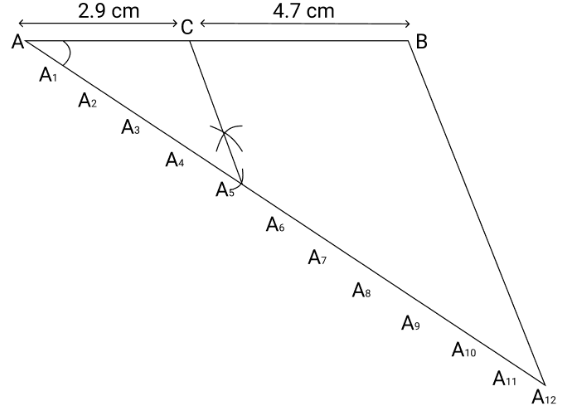Justification
The construction can be justified by proving that $\dfrac{{{\text{AC}}}}{{{\text{CB}}}} = \dfrac{5}{8}$

By construction, we have . By applying Basic proportionality theorem for the triangle ${\text{A}}{{\text{A}}_{13}}\;{\text{B}}$, we obtain

$\dfrac{{{\text{AC}}}}{{{\text{CB}}}} = \dfrac{{{\text{A}}{{\text{A}}_5}}}{{\;{{\text{A}}_5}\;{{\text{A}}_{13}}}} \ldots (1)$

From the figure, it can be observed that ${\text{A}}{{\text{A}}_5}$ and ${{\text{A}}_5}\;{{\text{A}}_{13}}$ contain 5 and 8 equal divisions of line segments respectively. $\dfrac{{{\text{A}}{{\text{A}}_5}}}{{\;{{\text{A}}_5}\;{{\text{A}}_{13}}}} = \dfrac{5}{8} \ldots$ (2)

On comparing equations (1) and (2), we obtain $\dfrac{{{\text{AC}}}}{{{\text{CB}}}} = \dfrac{5}{8}$

This justifies the construction.

2. Construct a triangle of sides 4 cm, 5cm and 6cm and then a triangle similar to it whose sides are $\dfrac{2}{3}$  of the corresponding sides of the first triangle. Give a proper justification of the construction.

Given: Construct a triangle of sides 4 cm, 5cm and 6cm and then a triangle similar to it whose sides are $\dfrac{2}{3}$ of the corresponding sides of the first triangle.

To find: Give a proper justification of the construction.

Step 1: Draw a line segment ${\text{AB}} = 4\;{\text{cm}}$. Taking point ${\text{A}}$ as centre, draw an arc of $5\;{\text{cm}}$ radius. Similarly, taking point B as its centre, draw an arc of $6\;{\text{cm}}$ radius. These arcs will intersect each other at point ${\text{C}}$. Now, ${\text{AC}} = 5\;{\text{cm}}$ and ${\text{BC}} = 6\;{\text{cm}}$ and $\Delta {\text{ABC}}$ is the required triangle.

Step 2: Draw a ray AX making an acute angle with line ${\text{AB}}$ on the opposite side of vertex ${\text{C}}$

Step 3: Locate 3 points ${{\text{A}}_1},\;{{\text{A}}_2},\;{{\text{A}}_3}($ as 3 is greater between 2 and 3) on line ${\text{AX}}$ such that ${\text{A}}{{\text{A}}_1} = {{\text{A}}_1}\;{{\text{A}}_2} =$ ${{\text{A}}_2}\;{{\text{A}}_3}$

Step 4: Join ${\text{B}}{{\text{A}}_3}$ and draw a line through ${{\text{A}}_2}$ parallel to ${\text{B}}{{\text{A}}_3}$ to intersect ${\text{AB}}$ at point ${\text{B}}$ '. Step 5. Draw a line through B' parallel to the line ${\text{BC}}$ to intersect ${\text{AC}}$ at ${\text{C}}$ '. $\vartriangle {\text{A}}{{\text{B}}^\prime }{{\text{C}}^\prime }$ is the required triangle.

The construction can be justified by proving that ${\text{A}}{{\text{B}}^\prime } = \dfrac{2}{3}{\text{AB}},{{\text{B}}^\prime }{{\text{C}}^\prime } = \dfrac{2}{3}{\text{BC}},{\text{A}}{{\text{C}}^\prime } = \dfrac{2}{3}{\text{AC}}$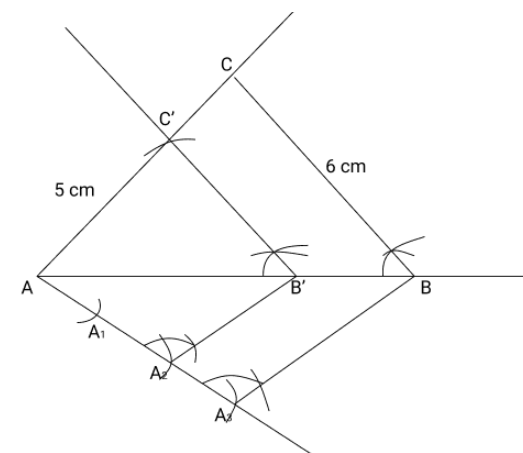By construction, we have

$\therefore \angle {\text{A}}{{\text{B}}^\prime }{{\text{C}}^\prime } = \angle {\text{ABC}}$ (Corresponding angles)

In $\vartriangle {\text{A}}{{\text{B}}^\prime }{{\text{C}}^\prime }$ and $\vartriangle {\text{ABC}}$,

$\angle {\text{ABC}} = \angle {\text{A}}{{\text{B}}^\prime }{\text{C}}$ (Proved above)

$\angle {\text{BAC}} = \angle {{\text{B}}^\prime }{\text{A}}{{\text{C}}^\prime }$ (Common)

$\therefore \vartriangle {\text{A}}{{\text{B}}^\prime }{{\text{C}}^\prime } \sim \vartriangle {\text{ABC}}$ (AA similarity criterion)

$\Rightarrow \dfrac{{{\text{A}}{{\text{B}}^\prime }}}{{{\text{AB}}}} = \dfrac{{{{\text{B}}^\prime }{{\text{C}}^\prime }}}{{{\text{BC}}}} = \dfrac{{{\text{A}}{{\text{C}}^\prime }}}{{{\text{AC}}}} \ldots \ldots$ (1)

In $\vartriangle {\text{A}}{{\text{A}}_2}\;{{\text{B}}^\prime }$ and $\vartriangle {\text{A}}{{\text{A}}_3}\;{\text{B}}$,

$\angle {{\text{A}}_2}{\text{A}}{{\text{B}}^\prime } = \angle {{\text{A}}_3}{\text{AB}}$ (Common)

$\angle {\text{A}}{{\text{A}}_2}\;{{\text{B}}^\prime } = \angle {\text{A}}{{\text{A}}_3}\;{\text{B}}$ (Corresponding angles)

$\therefore \vartriangle {\text{A}}{{\text{A}}_2}\;{{\text{B}}^\prime }$ and $\vartriangle {\text{A}}{{\text{A}}_3}\;{\text{B}}$ (AA similarity criterion)

$\Rightarrow \dfrac{{{\text{A}}{{\text{B}}^\prime }}}{{{\text{AB}}}} = \dfrac{{{\text{A}}{{\text{A}}_2}}}{{{\text{A}}{{\text{A}}_3}}}$

$\Rightarrow \dfrac{{{\text{A}}{{\text{B}}^\prime }}}{{{\text{AB}}}} = \dfrac{2}{3}\quad \ldots \ldots$

From equations (1) and (2),

$\dfrac{{{\text{A}}{{\text{B}}^\prime }}}{{{\text{AB}}}} = \dfrac{{{{\text{B}}^\prime }{{\text{C}}^\prime }}}{{{\text{BC}}}} = \dfrac{{{\text{A}}{{\text{C}}^\prime }}}{{{\text{AC}}}} = \dfrac{2}{3}$

$\Rightarrow {\text{A}}{{\text{B}}^\prime } = \dfrac{2}{3}{\text{AB}},{{\text{B}}^\prime }{{\text{C}}^\prime } = \dfrac{2}{3}{\text{BC}},{\text{A}}{{\text{C}}^\prime } = \dfrac{2}{3}{\text{AC}}$

This justifies the construction.

3. Construct a triangle with sides $5\;{\text{cm}},6\;{\text{cm}}$ and $7\;{\text{cm}}$ and then another triangle whose sides are $\dfrac{7}{5}$ of the corresponding sides of the first triangle. Give a proper justification of the construction.

Given: Construct a triangle with sides $5\;{\text{cm}},6\;{\text{cm}}$ and $7\;{\text{cm}}$ and then another triangle whose sides are $\dfrac{7}{5}$ of the corresponding sides of the first triangle.

To find:  Give a proper justification of the construction

Step 1: Draw a line segment ${\text{AB}}$ of $5\;{\text{cm}}$. Taking ${\text{A}}$ and ${\text{B}}$ as centre, draw arcs of $6\;{\text{cm}}$ and $5\;{\text{cm}}$ radius respectively. Let these arcs intersect each other at point C. $\vartriangle {\text{ABC}}$ is the required triangle having length of sides as $5\;{\text{cm}},6\;{\text{cm}}$, and $7\;{\text{cm}}$ respectively.

Step 2: Draw a ray AX making acute angle with line ${\text{AB}}$ on the opposite side of vertex ${\text{C}}$. Step 3. Locate 7 points, ${A_1},{A_2},{A_3},{A_4}{A_5},{A_6},{A_7}$ (as 7 is greater between 5 and 7 ), on line AX such that ${\text{A}}{{\text{A}}_1} = {{\text{A}}_1}\;{{\text{A}}_2} = {{\text{A}}_2}\;{{\text{A}}_3} = {{\text{A}}_3}\;{{\text{A}}_4} = {{\text{A}}_4}\;{{\text{A}}_5} = {{\text{A}}_5}\;{{\text{A}}_6} = {{\text{A}}_6}\;{{\text{A}}_7}$

Step 4: Join ${\text{B}}{{\text{A}}_5}$ and draw a line through ${{\text{A}}_7}$ parallel to ${\text{B}}{{\text{A}}_5}$ to intersect extended line segment ${\text{AB}}$ at point B'.

Step 5: Draw a line through B' parallel to ${\text{BC}}$ intersecting the extended line segment ${\text{AC}}$ at ${{\text{C}}^\prime }.\vartriangle {\text{A}}{{\text{B}}^\prime }{{\text{C}}^\prime }$ is the required triangle.Justification

The construction can be justified by proving that ${\text{A}}{{\text{B}}^\prime } = \dfrac{7}{5}{\text{AB}},{{\text{B}}^\prime }{{\text{C}}^\prime } = \dfrac{7}{5}{\text{BC}},{\text{A}}{{\text{C}}^\prime } = \dfrac{7}{5}{\text{AC}}$

In $\vartriangle {\text{ABC}}$ and $\vartriangle {\text{A}}{{\text{B}}^\prime }{{\text{C}}^\prime }$,

$\angle {\text{ABC}} = \angle {\text{A}}{{\text{B}}^\prime }{{\text{C}}^\prime }$ (Corresponding angles)

$\angle {\text{BAC}} = \angle {{\text{B}}^\prime }{\text{A}}{{\text{C}}^\prime }$ (Common)

$\therefore \vartriangle {\text{ABC}} \sim \Delta {\text{A}}{{\text{B}}^\prime }{{\text{C}}^\prime }$ (AA similarity criterion)

$\Rightarrow \dfrac{{{\text{AB}}}}{{{\text{A}}{{\text{B}}^\prime }}} = \dfrac{{{\text{BC}}}}{{{{\text{B}}^\prime }{{\text{C}}^\prime }}} = \dfrac{{{\text{AC}}}}{{{\text{A}}{{\text{C}}^\prime }}} \ldots (1)$

In $\vartriangle {\text{A}}{{\text{A}}_5}\;{\text{B}}$ and $\vartriangle {\text{A}}{{\text{A}}_7}\;{{\text{B}}^\prime }$,

$\angle {{\text{A}}_5}{\text{AB}} = \angle {{\text{A}}_7}{\text{A}}{{\text{B}}^\prime }$ (Common)

$\angle {\text{A}}{{\text{A}}_5}\;{\text{B}} = \angle {\text{A}}{{\text{A}}_7}\;{{\text{B}}^\prime }$ (Corresponding angles)

$\therefore \Delta {\text{A}}{{\text{A}}_5}\;{\text{B}} \sim \;\Delta {\text{A}}{{\text{A}}_7}\;{{\text{B}}^\prime }$ (AA similarity criterion)

$\Rightarrow \dfrac{{{\text{A}}{{\text{B}}^\prime }}}{{{\text{AB}}}} = \dfrac{{{\text{A}}{{\text{A}}_5}}}{{{\text{A}}{{\text{A}}_7}}}$

$\Rightarrow \dfrac{{{\text{AB}}}}{{{\text{A}}{{\text{B}}^\prime }}} = \dfrac{5}{7}\quad \ldots \ldots$

On comparing equations (1) and (2), we obtain $\dfrac{{{\text{AB}}}}{{{\text{A}}{{\text{B}}^\prime }}} = \dfrac{{{\text{BC}}}}{{{{\text{B}}^\prime }{{\text{C}}^\prime }}} = \dfrac{{{\text{AC}}}}{{{\text{A}}{{\text{C}}^\prime }}} = \dfrac{5}{7}$

$\Rightarrow {\text{A}}{{\text{B}}^\prime } = \dfrac{7}{5}{\text{AB}},{{\text{B}}^\prime }{{\text{C}}^\prime } = \dfrac{7}{5}{\text{BC}},{\text{A}}{{\text{C}}^\prime } = \dfrac{7}{5}{\text{AC}}$

This iustifies the construction.

4. Construct an isosceles triangle whose base is 8 cm and altitude 4 cm and then another triangle whose side are $1\dfrac{1}{2}$ times the corresponding sides of the isosceles triangle. Give a proper justification of the construction.

Given: Construct an isosceles triangle whose base is 8 cm and altitude 4 cm and then another triangle whose side are $1\dfrac{1}{2}$ times the corresponding sides of the isosceles triangle.

To find:  Give a proper justification of the construction.

Let us assume that $\Delta {\text{ABC}}$ is an isosceles triangle having CA and CB of equal lengths, base ${\text{AB}}$ of $8\;{\text{cm}}$, and ${\text{AD}}$ is the altitude of $4\;{\text{cm}}$.

A $\vartriangle {\text{A}}{{\text{B}}^\prime }{{\text{C}}^\prime }$ whose sides are $\dfrac{3}{2}$ times of $\Delta {\text{ABC}}$ can be drawn as follows.

Step 1: Draw a line segment ${\text{AB}}$ of $8\;{\text{cm}}$. Draw arcs of same radius on both sides of the line segment while taking point ${\text{A}}$ and ${\text{B}}$ as its centre. Let these arcs intersect each other at ${\text{O}}$ and ${{\text{O}}^\prime }$. Join ${\text{O}}{{\text{O}}^\prime }$. Let OO' intersect ${\text{AB}}$ at ${\text{D}}$

Step 2: Taking D as centre, draw an arc of $4\;{\text{cm}}$ radius which cuts the extended line segment ${\text{O}}{{\text{O}}^\prime }$ at point C. An isosceles $\vartriangle {\text{ABC}}$ is formed, having ${\text{CD}}$ (altitude) as $4\;{\text{cm}}$ and ${\text{AB}}$ (base) as $8\;{\text{cm}}$

Step 3: Draw a ray AX making an acute angle with line segment ${\text{AB}}$ on the opposite side of vertex ${\text{C}}$. Step 4. Locate 3 points (as 3 is greater between 3 and 2) ${{\text{A}}_1},\;{{\text{A}}_2}$, and ${{\text{A}}_3}$ on ${\text{AX}}$ such that ${\text{A}}{{\text{A}}_1} = {{\text{A}}_1}\;{{\text{A}}_2} =$ ${{\text{A}}_2}\;{{\text{A}}_3}$

Step 5: Join ${\text{B}}{{\text{A}}_2}$ and draw a line through ${{\text{A}}_3}$ parallel to ${\text{B}}{{\text{A}}_2}$ to intersect extended line segment ${\text{AB}}$ at point B'.

Step 6: Draw a line through B' parallel to BC intersecting the extended line segment ${\text{AC}}$ at ${{\text{C}}^\prime }.\vartriangle {\text{A}}{{\text{B}}^\prime }{{\text{C}}^\prime }$ is the required triangle.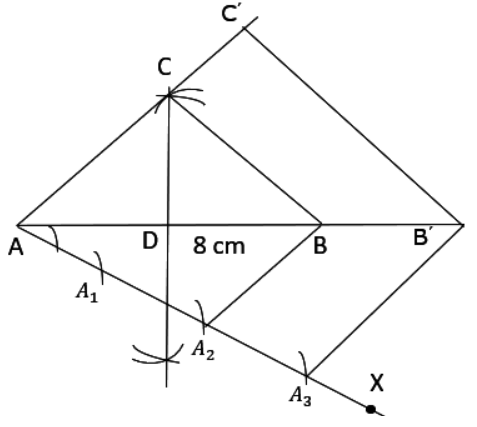Justification

The construction can be justified by proving that ${\text{A}}{{\text{B}}^\prime } = \dfrac{2}{3}{\text{AB}},{{\text{B}}^\prime }{{\text{C}}^\prime } = \dfrac{3}{2}{\text{BC}},{\text{A}}{{\text{C}}^\prime } = \dfrac{3}{2}{\text{AC}}$

In $\vartriangle {\text{ABC}}$ and $\Delta {\text{A}}{{\text{B}}^\prime }{{\text{C}}^\prime }$,

$\angle {\text{ABC}} = \angle {\text{A}}{{\text{B}}^\prime }{{\text{C}}^\prime }$ (Corresponding angles)

$\angle {\text{BAC}} = \angle {{\text{B}}^\prime }{\text{A}}{{\text{C}}^\prime }$ (Common)

$\therefore \vartriangle {\text{ABC}} \sim \Delta {\text{A}}{{\text{B}}^\prime }{{\text{C}}^\prime }$ (AA similarity criterion)

$\Rightarrow \dfrac{{{\text{AB}}}}{{{\text{A}}{{\text{B}}^\prime }}} = \dfrac{{{\text{BC}}}}{{{{\text{B}}^\prime }{{\text{C}}^\prime }}} = \dfrac{{{\text{AC}}}}{{{\text{A}}{{\text{C}}^\prime }}} \ldots (1)$

In $\Delta {\text{A}}{{\text{A}}_2}\;{\text{B}}$ and $\vartriangle {\text{A}}{{\text{A}}_3}\;{{\text{B}}^\prime }$

$\angle {{\text{A}}_2}{\text{AB}} = \angle {{\text{A}}_3}{\text{A}}{{\text{B}}^\prime }$ (Common)

$\angle {\text{A}}{{\text{A}}_2}\;{\text{B}} = \angle {\text{A}}{{\text{A}}_3}\;{{\text{B}}^\prime }$ (Corresponding angles)

$\therefore \Delta {\text{A}}{{\text{A}}_2}\;{\text{B}} \sim \vartriangle {\text{A}}{{\text{A}}_3}\;{{\text{B}}^\prime }$ (AA similarity criterion)

$\dfrac{{AB}}{{A{B^\prime }}} = \dfrac{{A{A_2}}}{{A{A_3}}} = \dfrac{2}{3}$

On comparing equations (1) and (2), we obtain $\dfrac{{{\text{AB}}}}{{{\text{A}}{{\text{B}}^\prime }}} = \dfrac{{{\text{BC}}}}{{{{\text{B}}^\prime }{{\text{C}}^\prime }}} = \dfrac{{{\text{AC}}}}{{{\text{A}}{{\text{C}}^\prime }}} = \dfrac{2}{3}$

$\Rightarrow {\text{A}}{{\text{B}}^\prime } = \dfrac{3}{2}{\text{AB}},{{\text{B}}^\prime }{{\text{C}}^\prime } = \dfrac{3}{2}{\text{BC}},{\text{A}}{{\text{C}}^\prime } = \dfrac{3}{2}{\text{AC}}$

This justifies the construction.

5. Draw a triangle ${\text{ABC}}$ with side ${\text{BC}} = 6\;{\text{cm}},{\text{AB}} = 5\;{\text{cm}}$ and $\angle {\text{ABC}} = {60^\circ }.$ Then construct a triangle whose sides are $\dfrac{3}{4}$ of the corresponding sides of the triangle ${\text{ABC}}$. Give a proper justification of the construction.

Given: Draw a triangle ${\text{ABC}}$ with side ${\text{BC}} = 6\;{\text{cm}},{\text{AB}} = 5\;{\text{cm}}$ and $\angle {\text{ABC}} = {60^\circ }.$ Then construct a triangle whose sides are $\dfrac{3}{4}$ of the corresponding sides of the triangle ${\text{ABC}}$

To find: Give a proper justification of the construction.

A $\Delta {{\text{A}}^\prime }{\text{B}}{{\text{C}}^\prime }$ whose sides are $\dfrac{3}{4}$ th of the corresponding sides of $\vartriangle {\text{ABC}}$ can be drawn as follows.

Step1: Draw a $\Delta {\text{ABC}}$ with side ${\text{BC}} = 6\;{\text{cm}},{\text{AB}} = 5\;{\text{cm}}$ and $\angle {\text{ABC}} = {60^\circ }$.

Step 2: Draw a ray BX making an acute angle with BC on the opposite side of vertex A.

Step 3: Locate 4 points (as 4 is greater in 3 and 4), ${{\text{B}}_1},\;{{\text{B}}_2},\;{{\text{B}}_3},\;{{\text{B}}_4}$, on line segment BX. Step 4. Join ${{\text{B}}_4}{\text{C}}$ and draw a line through ${{\text{B}}_3}$, parallel to ${{\text{B}}_4}{\text{C}}$ intersecting ${\text{BC}}$ at ${\text{C}}$ ' Step 5. Draw a line through C' parallel to AC intersecting ${\text{AB}}$ at ${{\text{A}}^\prime }.\Delta {{\text{A}}^\prime }{\text{B}}{{\text{C}}^\prime }$ is the required triangle.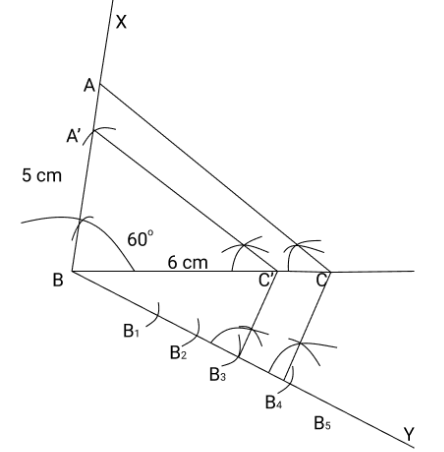Justification

The construction can be justified by proving ${\text{A}}{{\text{B}}^\prime } = \dfrac{3}{4}{\text{AB}},{\text{B}}{{\text{C}}^\prime } = \dfrac{3}{4}{\text{BC}},{{\text{A}}^\prime }{{\text{C}}^\prime } = \dfrac{3}{4}{\text{AC}}$

In $\vartriangle {{\text{A}}^\prime }{\text{B}}{{\text{C}}^\prime }$ and $\vartriangle {\text{ABC}}$,

$\angle {{\text{A}}^\prime }{{\text{C}}^\prime }{\text{B}} = \angle {\text{ACB}}$ (Corresponding angles)

$\angle {{\text{A}}^\prime }{\text{B}}{{\text{C}}^\prime } = \angle {\text{ABC}}$ (Common)

$\therefore \Delta {{\text{A}}^\prime }{\text{B}}{{\text{C}}^\prime } \sim \vartriangle {\text{ABC}}$ (AA similarity criterion)

$\Rightarrow \dfrac{{{{\text{A}}^\prime }{\text{B}}}}{{{\text{AB}}}} = \dfrac{{{\text{B}}{{\text{C}}^\prime }}}{{{\text{BC}}}} = \dfrac{{{{\text{A}}^\prime }{{\text{C}}^\prime }}}{{{\text{AC}}}} \ldots$ (1)

In $\Delta {\text{B}}{{\text{B}}_3}{{\text{C}}^\prime }$ and $\Delta {\text{B}}{{\text{B}}_4}{\text{C}}$,

$\angle {{\text{B}}_3}{\text{B}}{{\text{C}}^\prime } = \angle {{\text{B}}_4}{\text{BC}}$ (Common)

$\angle {\text{B}}{{\text{B}}_3}{{\text{C}}^\prime } = \angle {\text{B}}{{\text{B}}_4}{\text{C}}$ (Corresponding angles)

$\therefore \Delta {\text{B}}{{\text{B}}_3}{{\text{C}}^\prime } \sim \Delta {\text{B}}{{\text{B}}_4}{\text{C}}$ (AA similarity criterion)

$\Rightarrow \dfrac{{{\text{B}}{{\text{C}}^\prime }}}{{{\text{BC}}}} = \dfrac{{{\text{B}}{{\text{B}}_3}}}{{{\text{B}}{{\text{B}}_4}}}$

$\Rightarrow \dfrac{{{\text{B}}{{\text{C}}^\prime }}}{{{\text{B}}{{\text{C}}^\prime }}} = \dfrac{3}{4}\quad \ldots \ldots .(2)$

From equations (1) and (2), we obtain $\dfrac{{{{\text{A}}^\prime }{\text{B}}}}{{{\text{AB}}}} = \dfrac{{{\text{B}}{{\text{C}}^\prime }}}{{{\text{BC}}}} = \dfrac{{{{\text{A}}^\prime }{{\text{C}}^\prime }}}{{{\text{AC}}}} = \dfrac{3}{4}$

$\Rightarrow {{\text{A}}^\prime }{\text{B}} = \dfrac{3}{4}{\text{AB}},{\text{B}}{{\text{C}}^\prime } = \dfrac{3}{4}{\text{BC}},{\text{A}}{{\text{C}}^\prime } = \dfrac{3}{4}{\text{AC}}$

This justifies the construction.

6. Draw a triangle ${\text{ABC}}$ with side ${\text{BC}} = 7\;{\text{cm}},\angle {\text{B}} = {45^\circ },\angle {\text{A}} = {105^\circ }$. Then, construct a triangle whose sides are $4/3$ times the corresponding side of $\Delta {\text{ABC}}$. Give a proper justification of the construction.

Given: Draw a triangle ${\text{ABC}}$ with side ${\text{BC}} = 7\;{\text{cm}},\angle {\text{B}} = {45^\circ },\angle {\text{A}} = {105^\circ }$. Then, construct a triangle whose sides are $4/3$ times the corresponding side of $\Delta {\text{ABC}}$

To find: Give a proper justification of the construction.

$\angle {\text{B}} = {45^\circ },\angle {\text{A}} = {105^\circ }$

Sum of all interior angles in a triangle is ${180^\circ }$. $\angle {\text{A}} + \angle {\text{B}} + \angle {\text{C}} = {180^\circ }$

${105^\circ } + {45^\circ } + \angle {\text{C}} = {180^\circ }$

$\angle {\text{C}} = {180^\circ } - {150^\circ }$

$\angle {\text{C}} = {30^\circ }$

The required triangle can be drawn as follows.

Step 1: Draw a $\vartriangle {\text{ABC}}$ with side ${\text{BC}} = 7\;{\text{cm}},\angle {\text{B}} = {45^\circ },\angle {\text{C}} = {30^\circ }$.

Step 2: Draw a ray BX making an acute angle with ${\text{BC}}$ on the opposite side of vertex ${\text{A}}$. Step 3. Locate 4 points (as 4 is greater in 4 and 3), ${{\text{B}}_1},\;{{\text{B}}_2},\;{{\text{B}}_3},\;{{\text{B}}_4}$, on BX. Step 4. Join ${{\text{B}}_3}{\text{C}}$. Draw a line through ${{\text{B}}_4}$ parallel to ${{\text{B}}_3}{\text{C}}$ intersecting extended ${\text{BC}}$ at ${\text{C}}$ '. Step 5. Through ${{\text{C}}^\prime }$, draw a line parallel to ${\text{AC}}$ intersecting extended line segment at ${\text{C}}$. $\vartriangle {{\text{A}}^\prime }{\text{B}}{{\text{C}}^\prime }$ is the required triangle.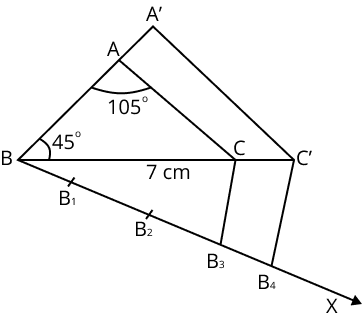Justification
The construction can be justified by proving that ${\text{A}}{{\text{B}}^\prime } = \dfrac{4}{3}{\text{AB}},{\text{B}}{{\text{C}}^\prime } = \dfrac{4}{3}{\text{BC}},{{\text{A}}^\prime }{{\text{C}}^\prime } = \dfrac{4}{3}{\text{AC}}$

In $\vartriangle {\text{ABC}}$ and $\vartriangle {{\text{A}}^\prime }{\text{B}}{{\text{C}}^\prime }$,

$\angle {\text{ABC}} = \angle {{\text{A}}^\prime }{\text{B}}{{\text{C}}^\prime }$ (Common)

$\angle {\text{ACB}} = \angle {{\text{A}}^\prime }{{\text{C}}^\prime }{\text{B}}$ (Corresponding angles)

$\therefore \Delta {\text{ABC}} \sim \Delta {{\text{A}}^\prime }{\text{B}}{{\text{C}}^\prime }$ (AA similarity criterion)

$\Rightarrow \dfrac{{{\text{AB}}}}{{{{\text{A}}^\prime }{\text{B}}}} = \dfrac{{{\text{BC}}}}{{{\text{B}}{{\text{C}}^\prime }}} = \dfrac{{{\text{AC}}}}{{{{\text{A}}^\prime }{{\text{C}}^\prime }}} \ldots$ (1)

In $\vartriangle {\text{B}}{{\text{B}}_3}{\text{C}}$ and $\vartriangle {\text{B}}{{\text{B}}_4}{{\text{C}}^\prime }$,

$\angle {{\text{B}}_3}{\text{BC}} = \angle {{\text{B}}_4}{\text{B}}{{\text{C}}^\prime }$ (Common)

$\angle {\text{B}}{{\text{B}}_3}{\text{C}} = \angle {\text{B}}{{\text{B}}_4}{{\text{C}}^\prime }$ (Corresponding angles)

$\therefore \angle {\text{B}}{{\text{B}}_3}{\text{C}} \sim \angle {\text{B}}{{\text{B}}_4}{{\text{C}}^\prime }$ (AA similarity criterion)

$\Rightarrow \dfrac{{{\text{BC}}}}{{{\text{B}}{{\text{C}}^\prime }}} = \dfrac{{{\text{B}}{{\text{B}}_3}}}{{{\text{B}}{{\text{B}}_4}}}$

$\Rightarrow \dfrac{{{\text{BC}}}}{{{\text{B}}{{\text{C}}^\prime }}} = \dfrac{3}{4}\quad \cdots \cdots$

On comparing equations (1) and (2), we obtain $\dfrac{{{\text{AB}}}}{{{{\text{A}}^\prime }{\text{B}}}} = \dfrac{{{\text{BC}}}}{{{\text{B}}{{\text{C}}^\prime }}} = \dfrac{{{\text{AC}}}}{{{{\text{A}}^\prime }{{\text{C}}^\prime }}} = \dfrac{3}{4}$

$\Rightarrow {{\text{A}}^\prime }{\text{B}} = \dfrac{4}{3}{\text{AB}},{\text{B}}{{\text{C}}^\prime } = \dfrac{4}{3}{\text{BC}},{{\text{A}}^\prime }{{\text{C}}^\prime } = \dfrac{4}{3}{\text{AC}}$

7. This justifies the construction.Draw a right triangle in which the sides (other than hypotenuse) are of lengths 4 cm and 3 cm. then construct another triangle whose sides are $\dfrac{5}{3}$ times the corresponding sides of the given triangle. Give the justification of the construction.

Given: Draw a right triangle in which the sides (other than hypotenuse) are of lengths 4 cm and 3 cm. then construct another triangle whose sides are $\dfrac{5}{3}$ times the corresponding sides of the given triangle.

To find: Give the justification of the construction,

It is given that sides other than hypotenuse are of lengths $4\;{\text{cm}}$ and $3\;{\text{cm}}.$ Clearly, these will be perpendicular to each other. The required triangle can be drawn as follows.

Step 1: Draw a line segment ${\text{AB}} = 4\;{\text{cm}}$. Draw a ray SA making ${90^\circ }$ with it.

Step 2: Draw an arc of $3\;{\text{cm}}$ radius while taking A as its centre to intersect ${\text{SA}}$ at ${\text{C}}$. Join ${\text{BC}}$. $\Delta {\text{ABC}}$ is the required triangle.

Step 3: Draw a ray AX making an acute angle with ${\text{AB}}$, opposite to vertex ${\text{C}}$

Step 4: Locate 5 points (as 5 is greater in 5 and 3 ), ${{\text{A}}_1},\;{{\text{A}}_2},\;{{\text{A}}_3},\;{{\text{A}}_4},\;{{\text{A}}_5}$, online segment ${\text{AX}}$ such that ${\text{A}}{{\text{A}}_1}$ $= {{\text{A}}_1}\;{{\text{A}}_2} = {{\text{A}}_2}\;{{\text{A}}_3} = {{\text{A}}_3}\;{{\text{A}}_4} = {{\text{A}}_4}\;{{\text{A}}_5}$

Step 5: Join ${{\text{A}}_3}\;{\text{B}}$. Draw a line through ${{\text{A}}_5}$ parallel to ${{\text{A}}_3}\;{\text{B}}$ intersecting extended line segment ${\text{AB}}$ at ${\text{B}}$ '. Step 6. Through B', draw a line parallel to BC intersecting extended line segment ${\text{AC}}$ at ${\text{C}}$ '. $\vartriangle {\text{A}}{{\text{B}}^\prime }{{\text{C}}^\prime }$ is the required triangle.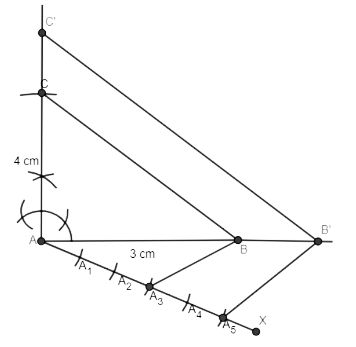Justification

The construction can be justified by proving that ${\text{A}}{{\text{B}}^\prime } = \dfrac{5}{3}{\text{AB}},{{\text{B}}^\prime }{{\text{C}}^\prime } = \dfrac{5}{3}{\text{BC}},{\text{A}}{{\text{C}}^\prime } = \dfrac{5}{3}{\text{AC}}$

In $\vartriangle {\text{ABC}}$ and $\vartriangle {\text{A}}{{\text{B}}^\prime }{\text{C}}$,

$\angle {\text{ABC}} = \angle {\text{A}}{{\text{B}}^\prime }{{\text{C}}^\prime }$ (Corresponding angles)

$\angle {\text{BAC}} = \angle {{\text{B}}^\prime }{\text{A}}{{\text{C}}^\prime }$ (Common)

$\therefore \vartriangle {\text{ABC}} \sim \vartriangle {\text{A}}{{\text{B}}^\prime }{{\text{C}}^\prime }$ (AA similarity criterion)

$\Rightarrow \dfrac{{{\text{AB}}}}{{{\text{A}}{{\text{B}}^\prime }}} = \dfrac{{{\text{BC}}}}{{{{\text{B}}^\prime }{{\text{C}}^\prime }}} = \dfrac{{{\text{AC}}}}{{{\text{A}}{{\text{C}}^\prime }}}\quad \cdots$

In $\vartriangle {\text{A}}{{\text{A}}_3}\;{\text{B}}$ and $\vartriangle {\text{A}}{{\text{A}}_5}\;{{\text{B}}^\prime }$,

$\angle {{\text{A}}_3}{\text{AB}} = \angle {{\text{A}}_5}{\text{A}}{{\text{B}}^\prime }$ (Common)

$\angle {\text{A}}{{\text{A}}_3}\;{\text{B}} = \angle {\text{A}}{{\text{A}}_5}\;{{\text{B}}^\prime }$ (Corresponding angles)

$\therefore \Delta {\text{A}}{{\text{A}}_3}\;{\text{B}} \sim \Delta {\text{A}}{{\text{A}}_5}\;{{\text{B}}^\prime }$ (AA similarity criterion)

$\Rightarrow \dfrac{{{\text{AB}}}}{{{\text{A}}{{\text{B}}^\prime }}} = \dfrac{{{\text{A}}{{\text{A}}_3}}}{{{\text{A}}{{\text{A}}_5}}}$

$\Rightarrow \dfrac{{{\text{AB}}}}{{{\text{A}}{{\text{B}}^\prime }}} = \dfrac{3}{5}$

On comparing equations (1) and (2), we obtain $\dfrac{{{\text{AB}}}}{{{\text{A}}{{\text{B}}^\prime }}} = \dfrac{{{\text{BC}}}}{{{{\text{B}}^\prime }{{\text{C}}^\prime }}} = \dfrac{{{\text{AC}}}}{{{\text{A}}{{\text{C}}^\prime }}} = \dfrac{3}{5}$

$\Rightarrow {\text{A}}{{\text{B}}^\prime } = \dfrac{5}{3}{\text{AB}},{{\text{B}}^\prime }{{\text{C}}^\prime } = \dfrac{5}{3}{\text{BC}},{\text{A}}{{\text{C}}^\prime } = \dfrac{5}{3}{\text{AC}}$

This justifies the construction.

EXERCISE NO: 11.2

1. Draw a circle of radius 6 cm. From a point 10 cm away from its centre, construct the pair of tangents to the circle and measure their lengths. Give a proper justification of the construction.

Given: Draw a circle of radius 6 cm. From a point 10 cm away from its centre, construct the pair of tangents to the circle and measure their lengths.

To prove: Give a proper justification of the construction.

A pair of tangents to the given circle can be constructed as follows.

Step 1: Taking any point O on the given plane as centre, draw a circle of 6 cm radius. Locate a point P, 10 cm away from O. Join OP.

Step 2: Bisect OP. Let M be the mid-point of PO.

Step 3: Taking M as centre and MO as radius, draw a circle.

Step 4: Let this circle intersect the previous circle at point Q and R.

Step 5: Join PQ and PR. PQ and PR are the required tangents.

The lengths of tangents PQ and PR are 8 cm each.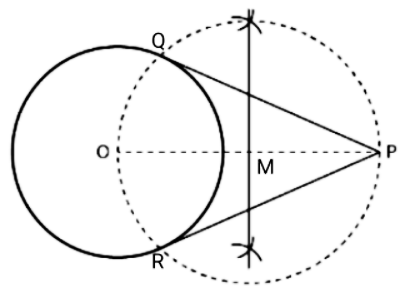JustificationThe construction can be justified by proving that PQ and PR are the tangents to the circle (whose centre is O and radius is 6 cm). For this, join OQ and OR.
∠PQO is an angle in the semi-circle. We know that the angle in a semicircle is a right angle.
$\therefore \angle PQO{\text{ }} = {\text{ }}90^\circ$

$\Rightarrow OQ \bot PQ$

Since OQ is the radius of the circle, PQ has to be a tangent of the circle. Similarly, PR is a tangent of the circle.

2. Construct a tangent to a circle of radius 4 cm from a point on the concentric circle of radius 6 cm and measure its length. Also verify the measurement by actual calculation. Give the justification of the construction.

Given: Construct a tangent to a circle of radius 4 cm from a point on the concentric circle of radius 6 cm and measure its length. Also verify the measurement by actual calculation.

To prove: Give the justification of the construction.

Tangents on the given circle can be drawn as follows.

Step 1: Draw a circle of 4 cm radius with centre as O on the given plane.

Step 2: Draw a circle of 6 cm radius taking O as its centre. Locate a point P on this circle and join OP.

Step 3: Bisect OP. Let M be the mid-point of PO.

Step 4: Taking M as its centre and MO as its radius, draw a circle. Let it intersect the given circle at the points Q and R.

Step 5: Join PQ and PR. PQ and PR are the required tangents.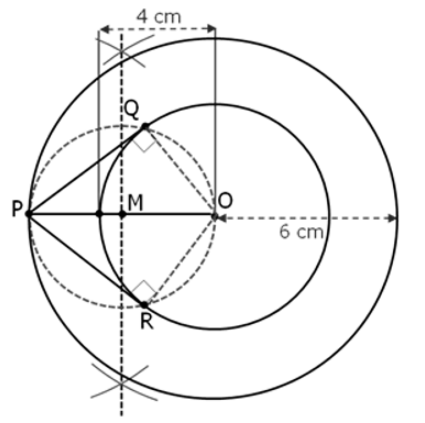It can be observed that PQ and PR are of length 4.47 cm each.
In $\Delta {\text{PQO}}$,

Since ${\text{PQ}}$ is a tangent, $\angle {\text{PQO}} = {90^\circ }$

${\text{PO}} = 6\;{\text{cm}}$

${\text{QO}} = 4\;{\text{cm}}$

Applying Pythagoras theorem in $\Delta {\text{PQO}}$, we obtain ${\text{P}}{{\text{Q}}^2} + {\text{Q}}{{\text{O}}^2} = {\text{P}}{{\text{Q}}^2}$

$P{Q^2} + {(4)^2} = {(6)^2}$

${\text{P}}{{\text{Q}}^2} + 16 = 36$

${\text{P}}{{\text{Q}}^2} = 36 - 16$

${\text{P}}{{\text{Q}}^2} = 20$

${\text{PQ}} = 2\sqrt 5$

${\text{PQ}} = 4.47\;{\text{cm}}$

Justification
The construction can be justified by proving that PQ and PR are the tangents to the circle (whose centre is O and radius is 4 cm). For this, let us join OQ and OR.$\angle PQO$ is an angle in the semi-circle. We know that the angle in a semicircle is a right angle.
$\therefore \angle PQO{\text{ }} = {\text{ }}90^\circ$

$\Rightarrow OQ \bot PQ$

Since OQ is the radius of the circle, PQ has to be a tangent of the circle. Similarly, PR is a tangent of the circle

3. Draw a circle of radius 3 cm. Take two points P and Q on one of its extended diameter each at a distance of 7 cm from its centre. Draw tangents to the circle from these two points P and Q. Give the justification of the construction.

Given: Draw a circle of radius 3 cm. Take two points P and Q on one of its extended diameter each at a distance of 7 cm from its centre. Draw tangents to the circle from these two points P and Q.

To prove: Give the justification of the construction.

The tangent can be constructed on the given circle as follows.

Step 1: Taking any point O on the given plane as centre, draw a circle of 3 cm radius.

Step 2: Take one of its diameters, PQ, and extend it on both sides. Locate two points on this diameter such that OR = OS = 7 cm.

Step 3: Bisect OR and OS. Let T and U be the mid-points of OR and OS respectively.

Step 4: Taking T and U as its centre and with TO and UO as radius, draw two circles. These two circles will intersect the circle at point V, W, X, Y respectively. Join RV, RW, SX, and SY. These are the required tangents.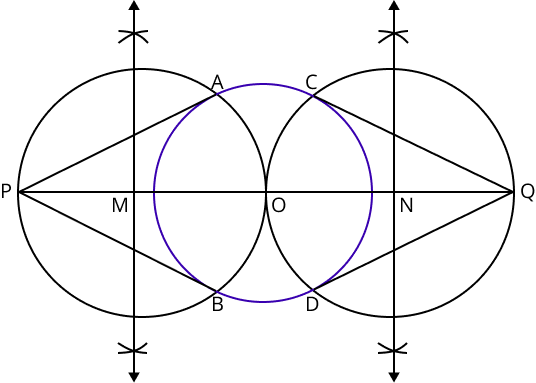Justification
The construction can be justified by proving that RV, RW, SY, and SX are the tangents to the circle (whose centre is O and radius is 3 cm). For this, join OV, OW, OX, and OY.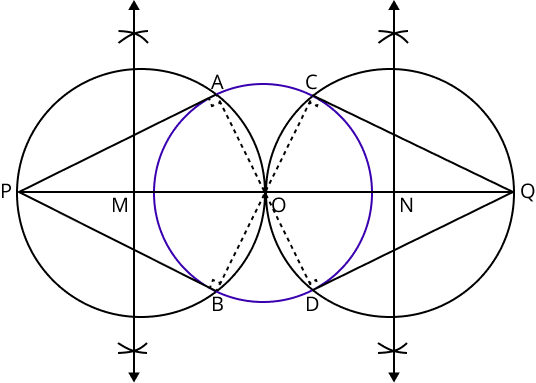$\angle RVO$is an angle in the semi-circle. We know that the angle in a semicircle is a right angle.
$\therefore \angle RVO{\text{ }} = {\text{ }}90^\circ$

$\Rightarrow OV \bot RV$

Since OV is the radius of the circle, RV has to be a tangent of the circle. Similarly, OW, OX, and OY are the tangents of the circle

4. Draw a pair of tangents to a circle of radius 5 cm which are inclined to each other at an angle of $60^\circ$. Give a proper justification of the construction.

Given: Draw a pair of tangents to a circle of radius 5 cm which are inclined to each other at an angle of $60^\circ$

To prove: Give a proper justification of the construction.

The tangents can be constructed in the following manner:

Step 1: Draw a circle of radius 5 cm and with centre as O.

Step 2: Take a point A on the circumference of the circle and join OA. Draw a perpendicular to OA at point A.

Step 3: Draw a radius OB, making an angle of $120^\circ {\text{ }}\left( {180^\circ {\text{ }} - {\text{ }}60^\circ } \right)$ with OA.

Step 4: Draw a perpendicular to OB at point B. Let both the perpendiculars intersect at point P. PA and PB are the required tangents at an angle of 60°.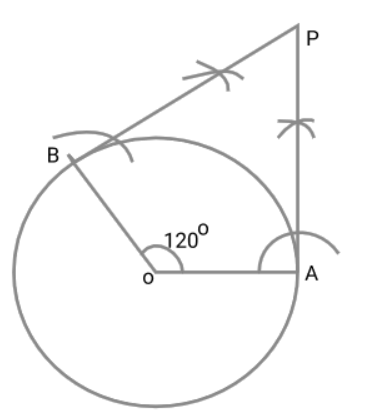Justification
The construction can be justified by proving that $\angle {\text{APB}} = {60^\circ }$ By our construction $\angle {\text{OAP}} = {90^\circ }$

$\angle {\text{OBP}} = {90^\circ }$

And $\angle {\text{AOB}} = {120^\circ }$

We know that the sum of all interior angles of a quadrilateral $= {360^\circ }$ $\angle {\text{OAP}} + \angle {\text{AOB}} + \angle {\text{OBP}} + \angle {\text{APB}} = {360^\circ }$

${90^\circ } + {120^\circ } + {90^\circ } + \angle {\text{APB}} = {360^\circ }$

$\angle {\text{APB}} = {60^\circ }$

This justifies the construction.

5. Draw a line segment AB of length 8 cm. Taking A as centre, draw a circle of radius 4 cm and taking B as centre, draw another circle of radius 3 cm. Construct tangents to each circle from the centre of the other circle. Give a proper justification for the construction.

Given: Draw a line segment AB of length 8 cm. Taking A as centre, draw a circle of radius 4 cm and taking B as centre, draw another circle of radius 3 cm. Construct tangents to each circle from the centre of the other circle.

To prove: Give a proper justification of the construction.

The tangents can be constructed on the given circles as follows.

Step 1: Draw a line segment AB of 8 cm. Taking A and B as centre, draw two circles of 4 cm and 3 cm radius.

Step 2: Bisect the line AB. Let the mid-point of AB be C. Taking C as centre, draw a circle of AC radius which will intersect the circles at points P, Q, R, and S. Join BP, BQ, AS, and AR. These are the required tangents.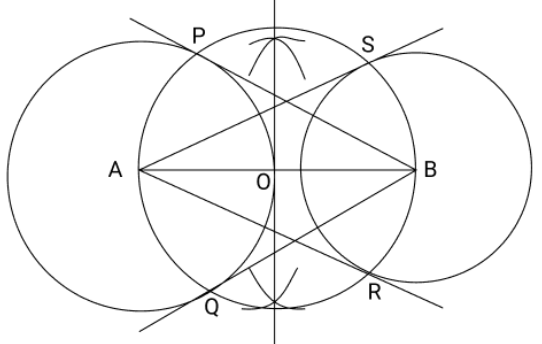Justification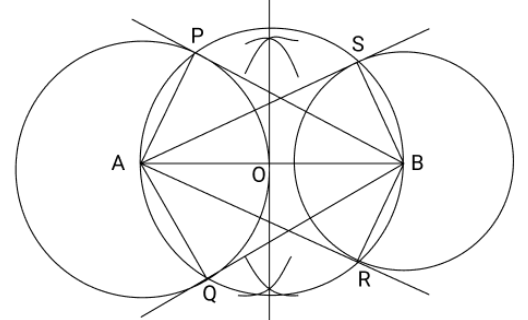The construction can be justified by proving that AS and AR are the tangents of the circle (whose centre is B and radius is 3 cm) and BP and BQ are the tangents of the circle (whose centre is A and radius is 4 cm). For this, join AP, AQ, BS, and BR.

$\angle ASB$ is an angle in the semi-circle. We know that an angle in a semicircle is a right angle.
$\therefore \angle ASB{\text{ }} = {\text{ }}90^\circ$

$\Rightarrow BS \bot AS$

Since BS is the radius of the circle, AS has to be a tangent of the circle. Similarly, AR, BP, and BQ are the tangents.

6. Let ABC be a right triangle in which AB = 6 cm, BC = 8 cm and $\angle B{\text{ }} = {\text{ }}90^\circ$. BD is the perpendicular from B on AC. The circle through B, C, and D is drawn. Construct the tangents from A to this circle. Give a proper justification of the construction.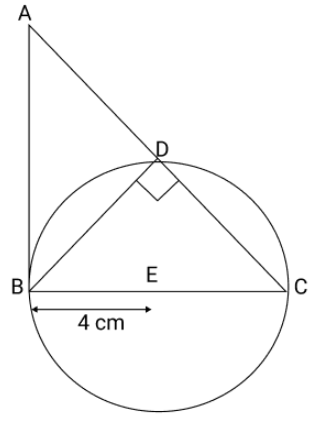Given: Let ABC be a right triangle in which AB = 6 cm, BC = 8 cm and $\angle B{\text{ }} = {\text{ }}90^\circ$. BD is the perpendicular from B on AC. The circle through B, C, and D is drawn. Construct the tangents from A to this circle.

To prove: Give a proper justification of the construction.

Consider the following situation. If a circle is drawn through B, D, and C, BC will be its diameter as $\angle BDC$ is of measure $90^\circ$. The centre E of this circle will be the midpoint of BC. The required tangents can be constructed on the given circle as follows.

Step 1: Join AE and bisect it. Let F be the mid-point of AE.

Step 2: Taking F as centre and FE as its radius, draw a circle which will intersect the circle at point B and G. Join AG. AB and AG are the required tangents.Justification
The construction can be justified by proving that AG and AB are the tangents to the circle. For this, join EG.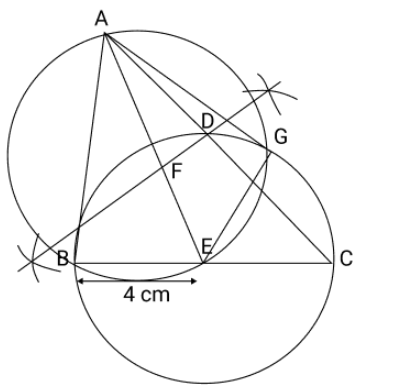$\angle AGE$ is an angle in the semi-circle. We know that an angle in a semicircle is a right angle.
$\therefore \angle AGE{\text{ }} = {\text{ }}90^\circ$

$\Rightarrow EG \bot AG$

Since EG is the radius of the circle, AG has to be a tangent of the circle.

$\angle B{\text{ }} = {\text{ }}90^\circ$

$\Rightarrow AB \bot BE$

Since BE is the radius of the circle, AB has to be a tangent of the circle.

7. Draw a circle with the help of a bangle. Take a point outside the circle. Construct the pair of tangents from this point to the circles. Give a proper justification of the construction.

Given: Draw a circle with the help of a bangle. Take a point outside the circle. Construct the pair of tangents from this point to the circles.

To prove: Give a proper justification of the construction.

The required tangents can be constructed on the given circle as follows.

Step 1: Draw a circle with the help of a bangle.

Step 2: Take a point P outside this circle and take two chords QR and ST.

Step 3: Draw perpendicular bisectors of these chords. Let them intersect each other at point O.

Step 4: Join PO and bisect it. Let U be the mid-point of PO. Taking U as centre, draw a circle of radius OU, which will intersect the circle at V and W. Join PV and PW.

PV and PW are the required tangents.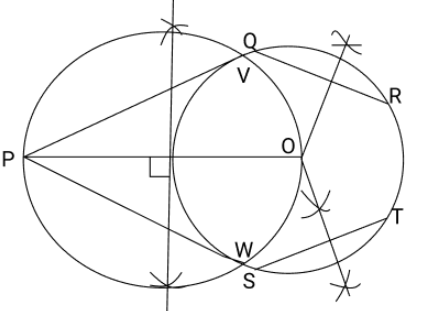Justification
The construction can be justified by proving that PV and PW are the tangents to the circle. For this, first of all, it has to be proved that O is the centre of the circle. Let us join OV and OW.We know that the perpendicular bisector of a chord passes through the centre.

Therefore, the perpendicular bisector of chords QR and ST pass through the centre.

It is clear that the intersection point of these perpendicular bisectors is the centre of the circle. $\angle PVO$ is an angle in the semi-circle. We know that an angle in a semicircle is a right angle.

$\therefore \angle PVO{\text{ }} = {\text{ }}90^\circ$

$\Rightarrow OV \bot PV$

Since OV is the radius of the circle, PV has to be a tangent of the circle. Similarly, PW is a tangent of the circle.

## NCERT Solutions for Class 10 Maths Chapter 11 Constructions - PDF Download

### Introduction

Construction of basic figures like a triangle, bisecting a line segment or drawing a perpendicular at a point on a line requires a ruler with a bevelled edge, a sharply pointed pencil preferably a type of set squares and a pair of compasses for justification of the method used. Some basic knowledge of geometry is required like proportionality theorem, concept of similar triangles, etc. have a look at to cover all the questions from exercise 11.1 and exercise 11.2

You can Find the Solutions of All the Maths Chapters below.

NCERT Solutions for Class 10 Maths

### Division of a Line Segment

To divide a line segment internally in a given ratio m:n, we take the following steps:

### Steps of Construction:

Step 1: Draw a line segment of a given length and name it AB.

Step 2: Draw a ray making an acute angle with AB and let the ray be AX.

Step 3: Along AX, mark off (m + n) points A1,  A2, ……., Am, Am+1,....., Am+n (for ex: if the ratio is to be 2:3, then we mark of 5 (= 2+ 3) points)

Step 4: Join BAAm+n.

Step 5: Draw a line through point Am parallel to Am+n B and make an angle equal to ∠AAm+n B.

Let this line meet Ab at a point P. Then this point is the required point which divides AB

internally in the ratio m:n.

### Constructing a Triangle Similar to a Given Triangle

Here we construct a triangle similar to a given triangle. The constructed triangle may be smaller or larger than the given triangle. So we define the following term:

Scale factor: Scale factor is the ratio of the sides of any figure to be constructed with the corresponding measurements of the given figure.

Let ABC be the given triangle by using the given & suppose we want to construct a triangle similar to ABC, such that each of its sides is (m/n)th of the corresponding sides of ABC.

### The Following Are the Steps to Be Taken for Construction a Triangle When M<n:

Step 1: Construct the given ABC by using the given data.

Step 2: Take AB as the base of the given ABC.

Step 3: At one end, say A of AB construct an acute angle ∠BAX below the base AB.

Step 4: Along AX mark off n points A1, A2,A3, ……., Am, Am+1,....., An such that AA1= A1A2 = A2A3

= A3 = Am-1Am = …….. = An-1An.

Step 5: Join AnB.

Step 6: Start from A & reach to the point AnB which meets AB at B’

Step 7: From B’ draw B’C’ || CB meeting AC at C’.

Then AB’C’ is the required triangle each of whose sides is (m/n)th of the corresponding sides of ABC.

### Construction of Tangents to a Circle

We know that when a point lies inside a circle no tangent can be drawn to the circle from this point. If a point lies on the circle at that point but if the point lies outside the circle, two agents can be drawn to the circle from the point.

### Case A: When the Centre of the Circle Is Known, the Steps of Construction Are:

Step 1: We take a point O on the plane of the paper and using a compass & ruler, we draw a circle of given radius.

Step 2: Let there be a point P on the circle.

Step 3: Join OP

Step 4: Construct ∠OPT = 90°.

Step 5: Produce TP to T’ to obtain the line TPT’ as the required tangent.

### Case B: When the Centre of the Circle is Not Known, Then the Steps of Construction Are:

Step 1: A chord PQ is drawn to the circle through the given point P on the circle.

Step 2: P & Q are joined to a point R in the major arc of the circle.

Step 3: Construct ∠QPT equal to ∠QRP on the opposite side of the chord PQ.

Step 4: Produce TP to T’ to obtain  TPT’ as the required tangent.

### Construction of Tangents to a Circle from an External Point

We know that two tangents can be drawn to a circle from an external point. The cases may arise---

### Case A: When the Centre of the Circle Is Known, the Steps of Construction Are:

Step 1: Given external point P is joined to centre O of the circle.

Step 2: Draw a perpendicular bisector of OP, intersecting OP at Q.

Step 3: Draw a circle with Q as center and OQ = QP as radius, intersecting the given circle at T & T’.

Step 4: Join PT & PT’.

Then PT & PT’ are the two tangents to the circle drawn from the external point P.

### Case B: When Centre of the Circle is Not Known, Then the Steps of Construction Are:

P is the external point & a circle is given with diameter AB.

Step 1: From P draw a secant PAB intersecting the given circle at A & B.

Step 2: Produce AP to C, such that AP = PC.

Step 3: Locate midpoint of BC as M, & draw a semicircle.

Step 4: Draw a perpendicular PD on BC intersecting the semicircle at D.

Step 5: With P as centre & radius PD draw arcs intersecting the given circle at T & T’.

Step 6: Join PT & PT’.

Then PT & PT’ are the two required tangents drawn on the given circle from the external point P

### Key Features for NCERT Solutions for Class 10 Maths Chapter 11 Constructions

The key features of the NCERT Class 10 Maths Chapter 11 Solutions as given below will help you to understand how useful these solutions are for your exam preparation.

• Step-by-step instructions for all constructions

• Prepared by subject experts

• Detailed explanations and reasoning for every sum

• Easy to access online and offline

• Available in PDF format

• Absolutely in accordance with the rules of construction for Class 10 Term 2 Maths

### Strategy for Preparing for Exams

For any exam, enough practice and revision are required. Even time management and how to tackle tricky questions are very significant during the exams. NCERT Solutions provided by Vedantu will definitely give you a good practice on the variety of questions. The notes along with the solution will give you clarity about the concept. Constructions is an important geometry chapter in Maths and pre knowledge of constructing an angle bisector and drawing a perpendicular line will be helpful. The NCERT Solutions design by Vedantu will give you a clear idea about the question papers that you will get in exams. The entire solution in Vedantu is designed in a concise manner and in step wise method. You can adopt the same method for exams. You will also learn how to manage time and deal with difficult questions in exams with the help of Vedantu’s NCERT Solutions.

### Advantage of using Vedantu’s NCERT Solution

There are many NCERT Solutions on the net but Vedantu provides 100% accurate and the latest solutions as per the syllabus under the strict guidelines set by the CBSE Board. Vedantu’s NCERT solutions have benefitted countless students till today. Experienced teachers have designed the solutions in a very simple way that makes it self explanatory and you will be thorough with the concepts at your fingertips. Vedantu also provides live sessions which is the highlight of Vedantu. This will boost the confidence of the students and will help master the subject. You can take the live video on any device and from anywhere.  For strengthening your concept understanding, you can completely rely on the NCERT Solutions provided by Vedantu. This is the right platform for you to revise your subjects and score more marks in exams. You can download the pdf format from Vedantu’s website which is available for free. You can download on any device and practice as per your convenient time. You can carry the pdf anywhere and anytime.

Vedantu offers the NCERT Solutions for Class 10 Maths Chapter 11 Constructions PDF for free on its mobile app and official website. Solving and practising these sums of Ex-11.1 and Ex.-11.2 will help students to enhance their understanding of the concepts of geometry covered in Class 10 CBSE Maths Term 2. Constructions of triangles, line segments, circles, tangents, etc. are covered in this chapter. Thus, the steps of the constructions explained in the NCERT Solutions Class 10 Maths Ch-11 will be of great help for their exam preparation.

Get the free PDF version of the solutions for this chapter. Learn how simply the experts have solved the geometry problems for your benefit. Focus on how to use the theories and construction methods explained in this chapter to score more in the exams.

## FAQs on NCERT Solutions for Class 10 Maths Chapter 11 - Constructions

1. What do you understand by the scale factor of any geometrical figure?

Scale factor is the ratio of the sides of any figure to be constructed with the corresponding measurements of the given figure.

2. Why should you refer to Vedantu for NCERT Solutions for Class 10 Chapter 11?

You should refer to Vedantu for NCERT Solutions for Class 10 Chapter 11 because Vedantu provides the latest and very comprehensive explanation of all NCERT Solution of Chapter 11. The experienced faculties in Vedantu have created the solutions just for you after intensive research. The solutions for Chapter 11 have many illustrated examples that will give you a complete revision of the chapter and you can prepare well for the exams. The NCERT Solutions provided by Vedantu are designed in a unique way starting from easy questions and as you proceed the level of the questions will become complicated. In this way you can solve any type of question whether it is easy or difficult.

3. What are the topics covered in the Chapter 11 for Class 10?

The topics that are covered in the Chapter 11 for Class 10 are how to determine a point dividing a line segment internally given a ratio, construction of similar triangles, constructing a tangent to a circle, and constructing a pair of tangents which are inclined to each other at an angle.

4. How can I improve my score through Vedantu?

Vedantu helps students to strengthen their foundation in all subjects and develop the ability to tackle different kinds of questions given in the textbooks. Free pdf of each subject topic wise  is available on the website. NCERT Maths book for class 10 guide will definitely help the students significantly to improve their performance in academics.

5. What is the basic concept of construction in Chapter 11 Constructions of Class 10 Maths?

A ruler with a bevelled edge, a highly pointed pencil, ideally a kind of set squares, and a pair of compasses for justification of the technique employed are required for the construction of fundamental figures such as a triangle, bisecting a line segment, or drawing a perpendicular at a point on a line. It is the basic concept of construction. Refer to Vedantu’s NCERT Solutions for Class 10 Chapter 11 to understand the concepts of this chapter.

6. What all constructions can be learnt in Chapter 11 Constructions of Class 10 Maths?

In this chapter, you'll learn how to determine a point by internally dividing a line segment given a ratio, how to construct similar triangles, how to construct a tangent to a circle, how to construct a pair of tangents, and how to construct a pair of tangents that are inclined to each other at an angle. You will learn more new definitions and interesting techniques in this chapter.

7. How much time do students need to complete Chapter 11 Constructions of Class 10 Maths?

There are only two exercises in Chapter 11 of Class 10 Maths. It will not take much time to do these exercises if your concepts are clear. Construction needs correct skills and patience. A lot of practice is required to do the construction in the right way. Vedantu offers NCERT Solutions for Class 10 Chapter 11 to help students understand the chapter better and score good marks in exams.

8. How many questions and examples are there in Chapter 11 Constructions of Class 10 Maths?

There are only two exercises in Chapter 11 of Class 10 Maths. Completing the exercises won’t take much time if your concepts are clear. So, there are 14 questions in and just two examples along with it to practise. Practicing all the questions honestly will be enough. If you have doubts, you can refer to NCERT Solutions for Class 10 Chapter 11 offered by Vedantu at free of cost available on the official website and on the Vedantu app. These solutions are prepared by experts in an easy to understand language and students can download them for free.

9. What should be the strategy for preparing Chapter 11 Constructions of Class 10 Maths?

A certain amount of practise and review is necessary for every exam. During the examinations, time management and how to approach difficult problems are also crucial. Vedantu's NCERT Solutions will offer you plenty of practise with a wide range of questions. The annotations, as well as the answer, will help you understand the idea. Construction is a crucial geometry subject in Math, and prior knowledge of how to create an angle bisector and draw a perpendicular line will come in handy.